# College Physics : Motion Diagrams

## Example Questions

### Example Question #1 : Motion Diagrams

The bases on a baseball field are 90 feet apart.

A players hits a home run and gets around the bases in 20 seconds what is the players total speed?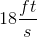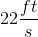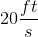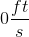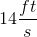Explanation: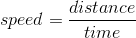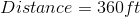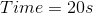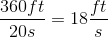### Example Question #1 : Motion Diagrams

The bases on a baseball field are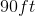apart in a perfect square.

A player hits a home run and goes around all 4 bases. What is the total distance he travelled?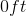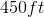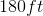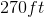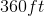Explanation:

There is 90 feet between each base and distance does not depend on direction. Distance is scaler therefore, the batter travels 360 feet.

### Example Question #1 : Motion Diagrams

The bases on a baseball field are 90 feet apart.

A players hits a home run and gets around the bases in 20 seconds what is the players total velocity?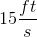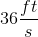Explanation: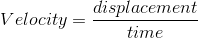Displacement is a vector. Magnitude and direction matter.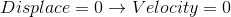### Example Question #1 : Motion Diagrams

The bases on a baseball field areapart.

A player hits a home run and runs around all four bases. What is his total displacement?Explanation:

Displacement is a vector. Therefore, magnitude and direction matters and because direction matters the total displacement is 0 feet.

### Example Question #11 : Motion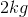ball is dropped and it hits the floor is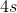. How high was the ball dropped from?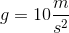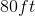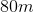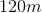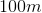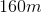Explanation:

We are dealing with motion in one plane and the initial velocity is zero. We must use the following kinematics equation to plug in known values and solve: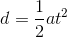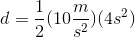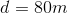### Example Question #31 : Mechanics

There arebetween the bases on a baseball field and they make a square.

Jim hits a double and reaches second base in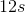. What is his velocity?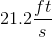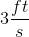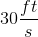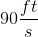Explanation: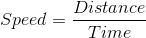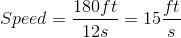Velocity is a vector so direction matters. Therefore this creates a right triangle with the two isosceles side having a velocity of. Therefore, the hypotenuse iswhen you perform Pythagorean theorem.

### All College Physics Resources